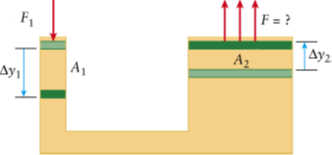# Problem: The hydraulic lift (the figure below) has a small piston with the surface  A1= 0.039 m2 and a large piston with the surface A2 = 5.0 m2. F1 = 21 N.  If the small piston is depressed a distance of Δy1, by how much will the large piston rise? (Express your answer to two significant figures.)

🤓 Based on our data, we think this question is relevant for Professor Willenbrock's class at UIUC.

###### Problem Details

The hydraulic lift (the figure below) has a small piston with the surface  A1= 0.039 m2 and a large piston with the surface A= 5.0 m2F= 21 N.  If the small piston is depressed a distance of Δy1, by how much will the large piston rise? (Express your answer to two significant figures.)